# M5-S5: Introduction to Circular Motion

• ### conduct investigations to explain and evaluate, for objects executing uniform circular motion, the relationships that exist between:

- centripetal
- mass
- speed

• Uniform circular motion is defined as when an object travels in a circular motion at constant speed. Hence, it does not experience acceleration in the direction of its rectilinear motion. However, it does experience acceleration in another direction due to centripetal force.

F_c=(mv^2)/r

Fc is the centripetal force of the circular motion, m is the mass (kg) of the object undergoing the motion, v (m/s) is the linear or tangential velocity of the object and r (m) is the radius of this circular motion.

Centripetal force provides the curvature to an object’s circular motion. Its direction is orthogonal (right-angled) to the direction of the object’s motion (velocity). In other words, it is always towards the centre of the circular motion. Centripetal acceleration (which can be calculated by Newton’s second law) is in the same direction as centripetal force.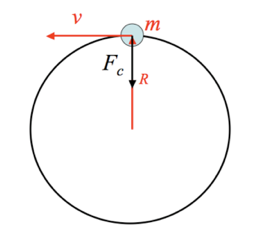### Centripetal force is a non-real force

This means that centripetal force always caused by a real force.

For example:

 ·       When an object with mass is attached to a rope and swung in circles, it undergoes circular motion. If the motion has constant velocity, the circulation motion is uniform. The centripetal force in this example is provided by the tension in the rope, which has a direction towards the centre of the circular motion.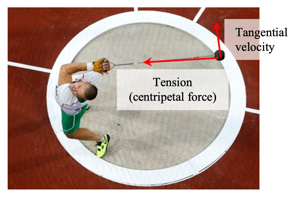·       In the case of a car travelling around a bend, it experiences centripetal force caused by kinetic friction between the car’s tyres and the ground.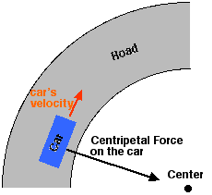·       When a spacecraft or satellite is orbiting the Earth, gravitational force provides the centripetal force for circular motion.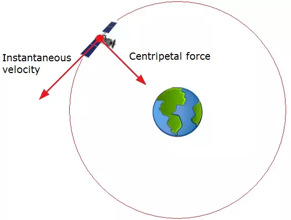### Relationship between centripetal force, mass, speed and radius

From the formula, we can deduce the following:

• Centripetal force is directly proportional to:
• Mass of the object undergoing circular motion
• Tangential velocity of the object squared
• Centripetal force is inversely proportional to:

Effect on centripetal force

• A stronger centripetal force is required to maintain an object’s uniform circular motion if:
• Its mass increases
• Its tangential velocity increases

 Change Centripetal force required to maintain circular motion Increase in mass Increase in centripetal force Increase in velocity Increase in centripetal force

Changes in centripetal force

• Conversely, changes in centripetal force can impact velocity and radius. Mass does not change.
• If centripetal force increases, velocity needs to increase in order to maintain the radius of circular motion, otherwise the object spirals inwards to reach a new circular path with smaller radius.
• If centripetal force decreases, velocity needs to decrease to maintain the radius of circular motion, otherwise the object spirals outwards to reach a new circular path with larger radius.

 Change Condition Effect Increase in centripetal force Velocity remains constant Radius decreases Increase in centripetal force Velocity increases Radius remains constant Decrease in centripetal force Velocity remains constant Radius increases Decrease in centripetal force Velocity decreases Radius remains constant

## Concept Question 1

A car rounds a bend on a road that follows the arc of a circle with radius r. The car has mass m and is travelling at a velocity v. Explain the following situations:

(a) Why are drivers advised to slow down during wet weather, specifically when they are making a bend.

(b) Assuming the friction between the tyres and the road does not change, describe the path of a car with mass 2m when it rounds the bend at velocity v?

(c) A motor cyclist rounds the same bend at velocity 2v. If the mass of the motorcycle is 0.25m, what would be different about the centripetal force acting on the motorcycle compared to that on the car?

## Concept Question 2

HSC Q30 2013

﻿The diagram shows a futuristic space station designed to simulate gravity in a weightless environment.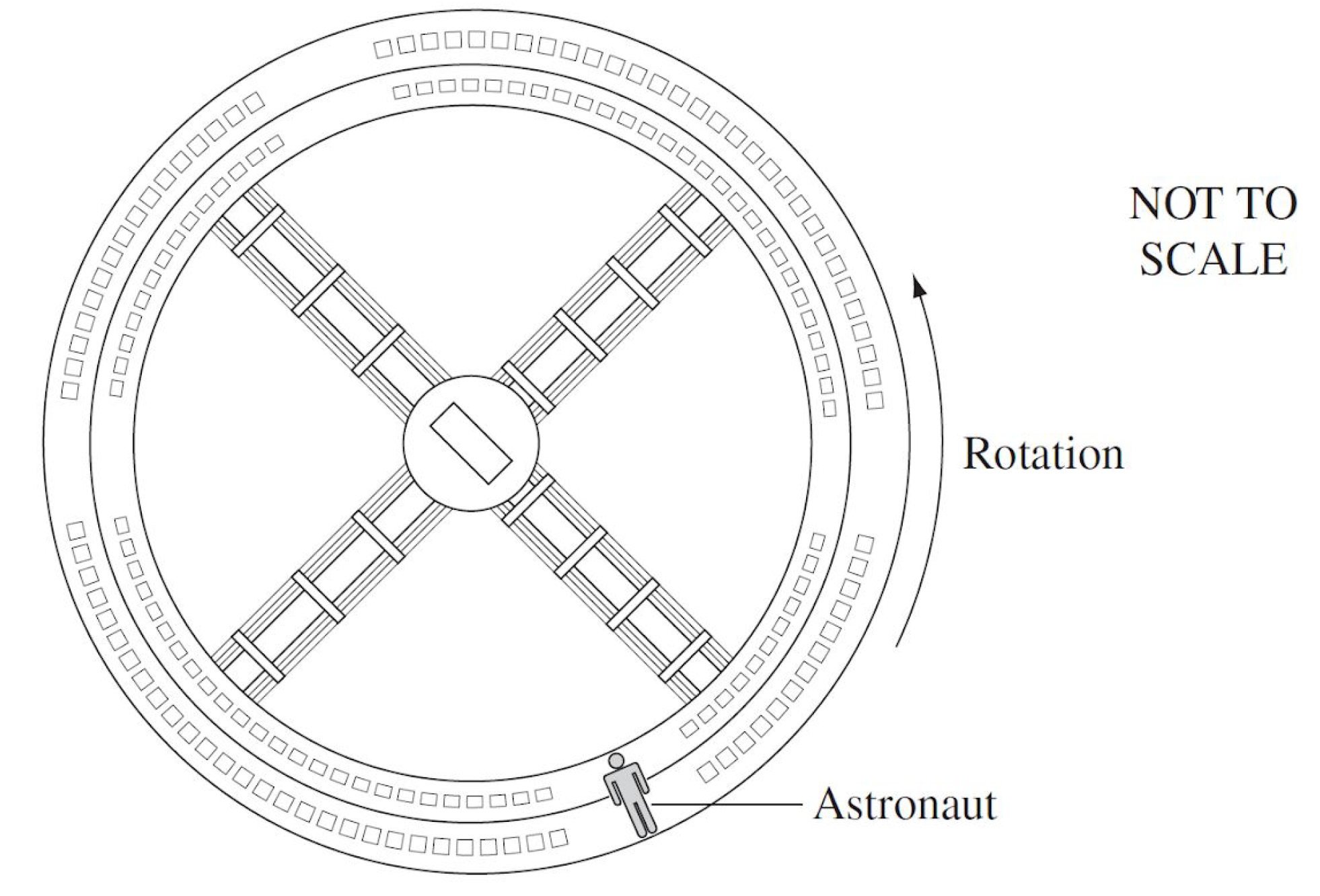(a) Explain how the rotating space station simulates gravity.

(b) Describe the effect of the space station’s rotation speed on the magnitude of centripetal force.

(c) To maintain ‘gravity’, does the space station’s rotation speed need to change if its radius changes? Explain your answer.

(d) Does the mass of the space station influence the rotation speed required to simulate gravity? Justify your answer.

## Concept Questions Solutions

Question 1

(a) During wet weather, the kinetic friction between a car's tyres and the ground is reduced. This means the centripetal force acting on the car during its bend is reduced. As a result, velocity needs to decrease to maintain radius of the curvature.

(b) Since centripetal force remains constant, the radius of curvature is doubled. This means for a car with mass 2m travelling at the same speed v, it requires a greater distance to complete the bend.

(c)

F_c=(0.25m(2v)^2)/r

F_c=(0.25m*4v^2)/r

F_c=(mv^2)/r

As seen above, substituting 0.25m and 2v into the equation F_c=(mv^2)/r will yield a magnitude of centripetal force identical to one with mass m and velocity v. This means the centripetal force acting on the motorcycle remains unchanged and so does its radius.

Question 2

(a) The rotating motion of the spacecraft exerts normal force (centripetal force) on the astronaut. Due to Newton's third law, the astronaut exerts reaction force on the outer perimeter of the spacecraft. The acceleration resulted from this reaction force simulates gravity.

(b) As v increases, the magnitude of centripetal force increases (since radius remains constant). Changes in centripetal force are always proportional to the square of change in velocity.

(c) ﻿Yes, to maintain gravity, the magnitude of centripetal force cannot be changed. A decrease in radius needs to be compensated by a reduction in velocity v. This means the rotational speed to simulate gravity is lower for smaller space stations.

﻿(d) No, the mass of the space station does not affect centripetal force nor acceleration. In addition, the mass of the astronaut does not influence the rotational speed required to achieve ﻿1g﻿ of gravitational acceleration. This is because simulated gravity is independent of mass: a_c=v^2/r.